# 11 Two slopes method

For a description of the two slopes method see .
This method is shown unless the software was compiled without Fortran support.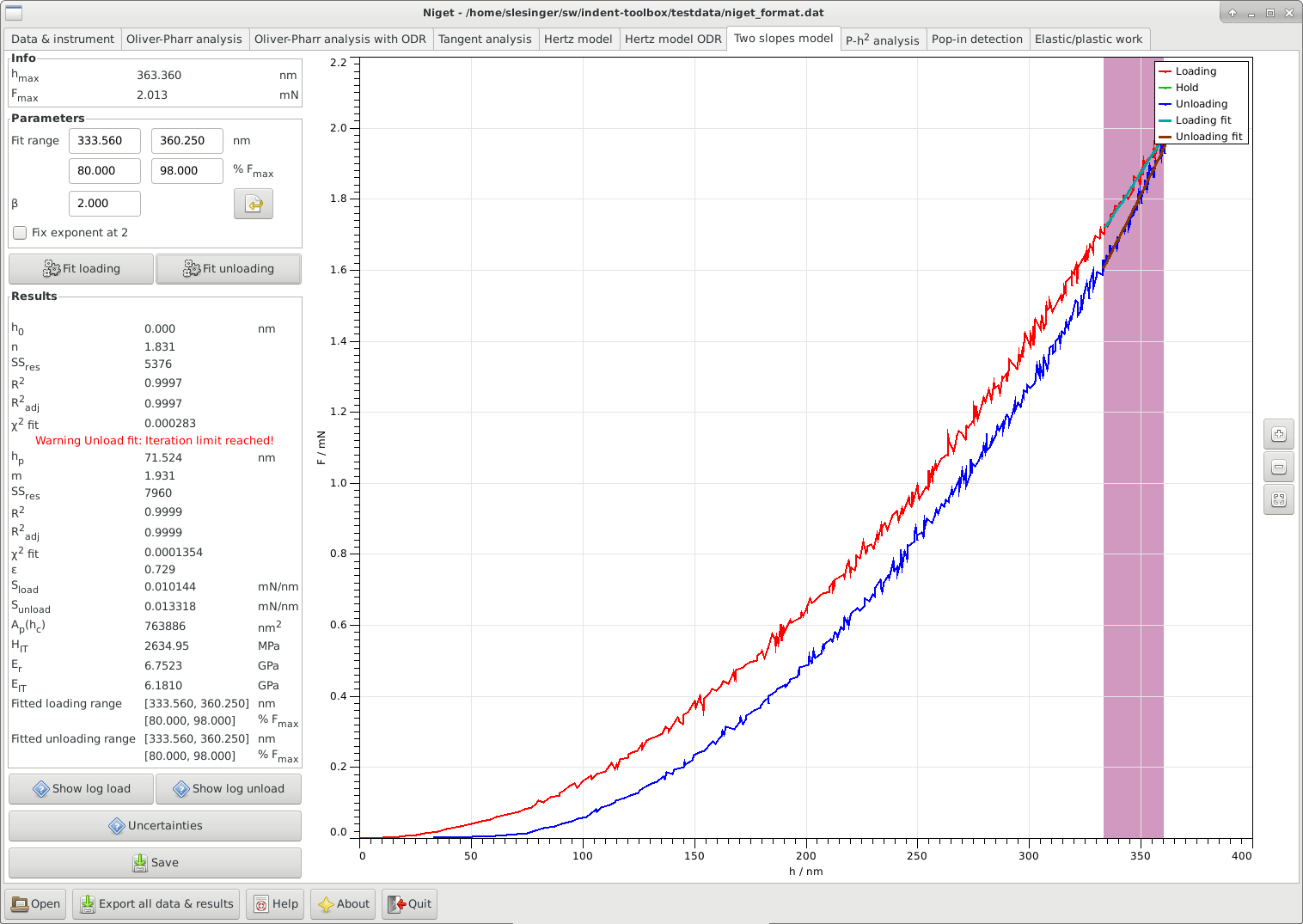Figure 11: Two slopes analysis using orthogonal data regression

## 11.1 Window

The window consists of several blocks:

• Info displays the maximum depth and force during the indentation

• Parameters shows the selected range in nm and in % of the maximum force, and the correction.

• -

The fitting range can be selected either using the mouse or typing in the range entries. The range can be defined either in nm or in percent of the maximum force. It is often recommended to use the range 80–98 % F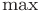for the fit, see section 11.2.

• -

The parameteraccounts for any deviations from the axisymmetric case and is used in the calculation of the reduced modulus in equation (10). Currently, the default value is no correction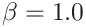. The value supplied by the user is saved in the settings and can be reset to its default value.

• -

Check box, whether or not the exponent of the loading curve should be fitted or fixed at the theoretical value 2, see 11.2.

• Results displays all results in the following order: the auxiliary depth parameter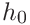, the powerof the power law loading function, the residual depth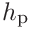, the power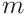of the power law unloading function, the parameter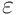, the loading slope, the unloadingslope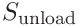, the contact area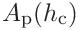, the indentation hardness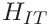, the contact modulus, the indentation modulus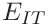and the ranges used for the fitting procedures. The variables are described in detail in section 11.2. Warnings are displayed if the fittings procedures failed.

• Uncertainties show the uncertainty analysis window, see section 15.2.4.

• Save save parameters and results to given file.

• Graph display the unloading curve and the fitted curves. Stepwise zooming/unzooming can be performed by selecting a range with the mouse and pressing the Zoom/ Unzoom buttons. The graph is restored to its original size by the Restore button.

## 11.2 Procedure

The two slopes method combines the standard Oliver Pharr method and the quadratic loading curve to avoid the need of the contact area.

1. 1.

Fit the upper part of the loading curve with a power law functionusing orthogonal least squares as implemented in the package ODRPACK95 . The range should be approx. 85–98 % F. The exponentcan be kept fixed at its theoretical value 2.

2. 2.

Fit the upper part of the unloading curve with a power law functionusing orthogonal least squares as implemented in the package ODRPACK95 . The range should be approx. 85–98 % F. All three parameters are fitted.

3. 3.

The auxiliary parameteris calculated from the poweras in (6)

4. 4.

The slopes at the maximum depth are calculated as(23)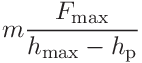(24)
5. 5.

The contact area, indentation hardness and contact modulus are calculated as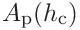(25)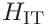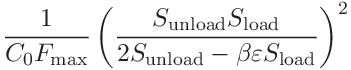(26)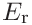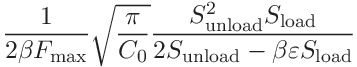(27)

with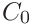the coefficient of the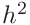term in the area calibration function anda geometric correction term. Currently, we use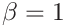.

6. 6.

The indentation modulus can be calculated from (11)